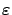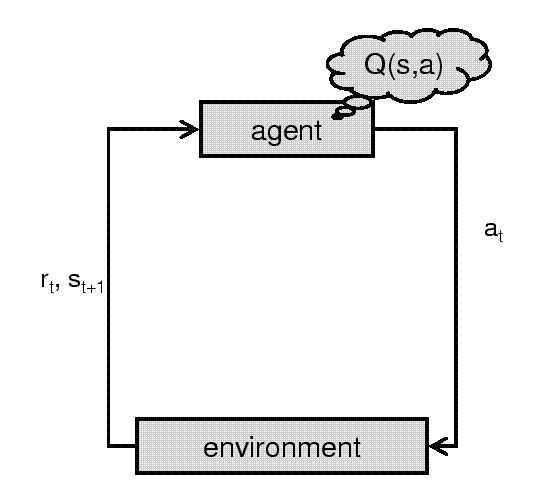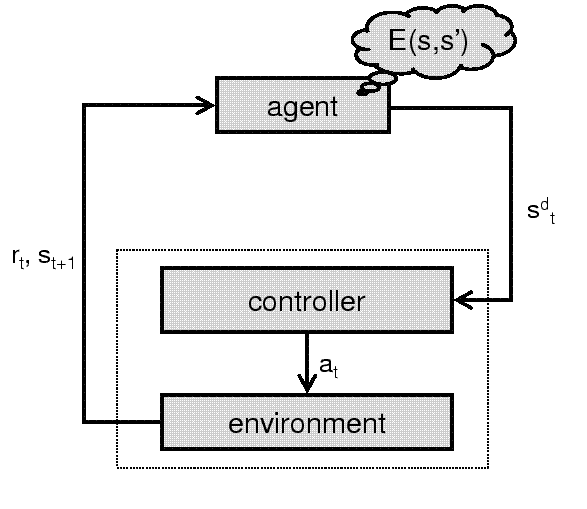Next: Formal Description of Event-learning Up: -MDPs: Learning in Varying Previous: Q-learning in Generalized -MDPs

# Illustration: the Event-learning Algorithm

Event-learning is a novel learning algorithm, where optimality can be proved by the generalized-MDP theory. Those readers who are familiar with event-learning can skip the following introductory material and go directly to Section 4.2.

Most reinforcement learning algorithms learn policies that are mappings from states to actions, that is, they are telling which action to take in a given state. The idea behind event-learning (E-learning,3 for short) is that the agent should learn a policy that (in a given state) indicates a new target state. The target is then approached by a lower-level controller. This setting is natural in real physical environments, but also applicable in non-physical problems by defining a simple controller. For example, in a labyrinth, the controller may know that it can get from cell (2,3) to (2,4) by the action south'. (The controller can be a dummy'; it does not need to know the path between two distant states.)

Using a lower-level controller means that the agent learns subgoals' it should select instead of learning actions'. In event-learning, desired subgoals can be seen as `actions'. Naturally, a subgoal is useful only if (1) it leads toward the overall goal and (2) the controller can accomplish this subgoal with high probability. A major advantage of this approach is that it can be made robust against changes in the environment: actions may change considerably to accommodate changes of the environment, while learned subgoal sequences may remain valid. The concept of E-learning is compared to classical policy iteration in Figure 1 and 2.In this section we briefly overview the event-learning scheme. First, we formalize the problem, then we introduce the E-learning algorithm and a robust controller. After this, we apply the theory of generalized-MDPs to obtain theoretical performance guarantees for our algorithm. Finally, we provide some computer simulations to show the practical utility of our approach.

SubsectionsNext: Formal Description of Event-learning Up: -MDPs: Learning in Varying Previous: Q-learning in Generalized -MDPs